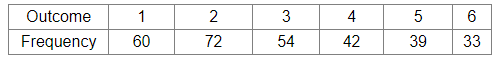# A dice is thrown 300 times and the outcomes are noted as given below.Question:

A dice is thrown 300 times and the outcomes are noted as given below.When a dice is thrown at random, what is the probability of getting a
(i) 3?
(ii) 6?
(iii) 5?
(iv) 1?

Solution:

Total number of throws =  300
In a random throw of a dice, let E1, E2, E3, E4, be the events of getting 3, 6, 5 and 1, respectively. Then

(i) $P($ getting 3$)=P\left(E_{1}\right)=\frac{\text { Number of times } 3 \text { appears }}{\text { Total number of trials }}=\frac{54}{300}=0.18$

(ii) $P$ (getting 6 ) $=P\left(E_{2}\right)=\frac{\text { Number of times } 6 \text { appears }}{\text { Total number of trials }}=\frac{33}{300}=0.11$

(iii) $P($ getting 5$)=P\left(E_{3}\right)=\frac{\text { Number of times } 5 \text { appears }}{\text { Total number of tr ials }}=\frac{39}{300}=0.13$

(iv) $P$ (getting 1) $=P\left(E_{4}\right)=\frac{\text { Number of times 1 appears }}{\text { Total number of trials }}=\frac{60}{300}=0.20$